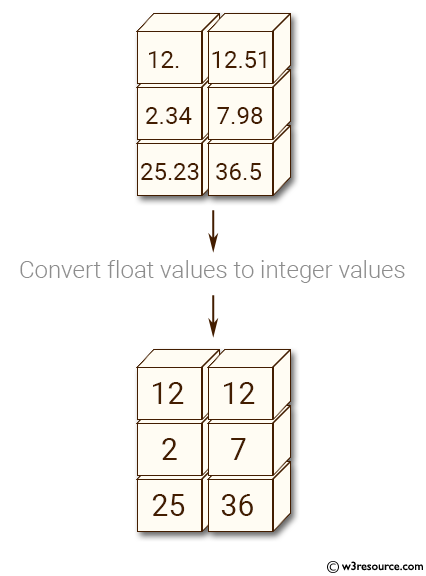﻿ NumPy: Convert a NumPy array of float values to a NumPy array of integer values - w3resource# NumPy: Convert a NumPy array of float values to a NumPy array of integer values

## NumPy: Array Object Exercise-82 with Solution

Write a NumPy program to convert a NumPy array of float values to a NumPy array of integer values.

Pictorial Presentation:Sample Solution:-

Python Code:

``````import numpy as np
x= np.array([[12.0, 12.51], [2.34, 7.98], [25.23, 36.50]])
print("Original array elements:")
print(x)
print("Convert float values to integer values:")
print(x.astype(int))
```
```

Sample Output:

```Original array elements:
[[ 12.    12.51]
[  2.34   7.98]
[ 25.23  36.5 ]]
Convert float values to integer values:
[[12 12]
[ 2  7]
[25 36]]
```

Python Code Editor:

Have another way to solve this solution? Contribute your code (and comments) through Disqus.

What is the difficulty level of this exercise?

Test your Python skills with w3resource's quiz

﻿

## Python: Tips of the Day

List comprehension with the current and previous value:

```>>> a = [1, 2, 4,2]
>>> [y - x for x,y in zip(a,a[1:])]
[1, 2, -2]
```